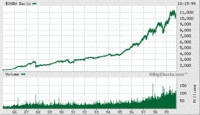# Using functions in an introductory geoscience course

## Summary

This is a template and set of exercises designed to helpfaculty increase the graphical literacy of their students. The template gives general guidelines for teaching students the relationship between functions and their graphical representation. Population growth and atmospheric CO2 increase are two exercises that are included to help teach exponential growth and decay. The template and exercises guide faculty through helping students to make connections between datasets, graphs and functions.

Used this activity? Share your experiences and modifications

## Learning Goals

• use data to plot a curve or a line
• read information from a plot
• recognize different functions, in numerical, graphical, and algebraic forms
• calculate slopes of functions
• interpolation and extrapolation
• (optional) learning to use appropriate technology (e.g., Excel)

## Context for Use

The template is appropriate for use in teaching functions in introductory geology. These exercises can be used as a homework assignment or can be used during lecture to walk students through graphing and functions.

## Description and Teaching Materials

1. Template for teaching functions (Acrobat (PDF) 144kB Jan20 04) with guidelines for helping students to make connections,
2. Exercise dealing with population growth (Acrobat (PDF) 160kB Jan20 04) and exponential functions,
3. Exercise dealing with exponential functions in relation to atmospheric carbon dioxide increase (Acrobat (PDF) 152kB Jan20 04) through time.

## Teaching Notes and Tips

We envision this template as an outline for teachers of introductory geology classes (physical, historical, environmental, etc.) who want to develop the graphical literacy of their students. They will be able to adapt this template for any graph or function of interest. Similarly, it can be adapted to illustrate functions in a pre-calculus course.

We guide you through reasoning for giving students CONTEXT, showing them and guiding them through exploring DATA, preparing students to GRAPH, exploring the GRAPHICAL expression, introduction and use of EQUATION, and ANALYSIS. Each graph will be different but we also encourage you to have students to think deeply about the mathematics and geology behind what you have shown the students by asking thoughtful questions.

## Assessment

These exercises are most easily assessed by giving them as homework and grading them.

However, the template may be used as a part of a lecture and if this is the case, questions on an exam in which students must interpret a graph or choose the equation that is most like its graphical representation may be appropriate.

## References and Resources

### Functions

• Wolfram has an entire site dedicated to functions. They have information on all types of functions from elementary to elliptical integrals to polylogarithmic functions.